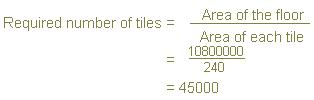# Class 8, CBSE Math Guide | Solutions of NCERT Mathematics Exercise 11.1 Mensuration

CBSE Board, Class VIII Mathematics
Chapter 11 Mensuration
Ncert Solutions of Mathematics Textbook Exercise 11.1 (Page 171)
Q.1: A square and a rectangular field with measurements as given in the figure have the same perimeter. Which field has a larger area?Solution:
(a) Perimeter of square = 4 (Side of the square) = 4 x 60 = 240 m
(b) Perimeter of rectangle = 2 (Length + Breadth) = 2 (80 + Breadth)
or, 240 = 2 (80 + Breadth)
or, 120 = 80 + Breadth
Area of square = (Side)2 = (60 m)2 = 3600 m2
Area of rectangle = Length × Breadth = 80 m × 40 m = 3200 m2
Hence, square field has larger area than the area of the rectangular field.

Q.2: Mrs. Kaushik has a square plot with the measurement as shown in the following figure. She wants to construct a house in the middle of the plot. A garden is developed around the house. Find the total cost of developing a garden around the house at the rate of Rs 55 per m2.Solution:
Area of garden = Area of square plot – Area of rectangular plot (middle plot)
= (25)2 – (20 x 15) = 325 m2
The total cost of developing garden around the house at the rate of Rs 55 per m2 = 55 × 325 = Rs 17,875

Q. 3: The shape of a garden is rectangular in the middle and semi circular at the ends as shown in the diagram. Find the area and the perimeter of the garden.Solution:
Length of the rectangle = 20 – (3.5 + 3.5) = 13 m
Breadth of the rectangle = 7 m
Radius of the semi-circle = 3.5 m
We know two semi-circle = one circle
Hence, perimeter of the circle = 2 π r
= 2 x 22/7 x 3.5 = 22 m
Perimeter of the rectangle = 2 (l + b)
= 2 (13 + 7) = 40 m
Hence, perimeter of the garden = (22 m + 40 m) = 62 m
Now, area of the rectangle = 13 x 7 = 91 m2
Area of the circle (two semi-circles) = π r2 22/7 x 3.5 x 3.5 = 38.5 m2
Hence, Area of garden = 38.5 m2 + 91 m2 = 129.5 m2

Q. 4: A flooring tile has the shape of a parallelogram whose base is 24 cm and the corresponding height is 10 cm. How many such tiles are required to cover a floor of area 1080 m2? (If required you can split the tiles in whatever way you want to fill up the corners).

Solution:
Area of parallelogram = base × height
So, area of each tile = 24 × 10 = 240 cm2
Area of the floor = 1080 m2 = (1080 x 100 x 100) cm2Q. 5: An ant is moving around a few food pieces of different shapes scattered on the floor. For which food − piece would the ant have to take a longer round? Remember, circumference of a circle can be obtained by using the expression c = 2πr, where r is the radius of the circle.Solution:Therefore, the ant will have to take a longer round for the food-piece (b), because the perimeter of the figure given in alternative (b) is the greatest among all.

1.can u plz arrange 4 a sample paper of math for gr8?
by tomorrow...n yeah...this site is awesomeeeee!!!!

2.Anshita, thanks for writing in.
Genuine requests as of yours, are often acceded here. Next time please allow us at least 2 - 3 days so that we can meet your requirement ..

3.thats cool:)

4.Superbbbbbbbbb 😀😀😀😀😀😀😀😀

5.Superbbbbbbbbb 😀😀😀😀😀😀😀😀

6.